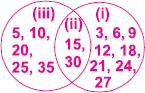# User ForumSubject :IMO    Class : Class 4

The numbers in region (ii) shows that the number can be divided by _____ both.A 5 and 3
B 15 and 9
C 20 and 9
D 5 and 20

## Ans 1:

Class : Class 7
The answer is A because all the other numbers are the multiples of 3 and 5

Class : Class 7
15 and 30 also

Class : Class 7
and 15,30 also